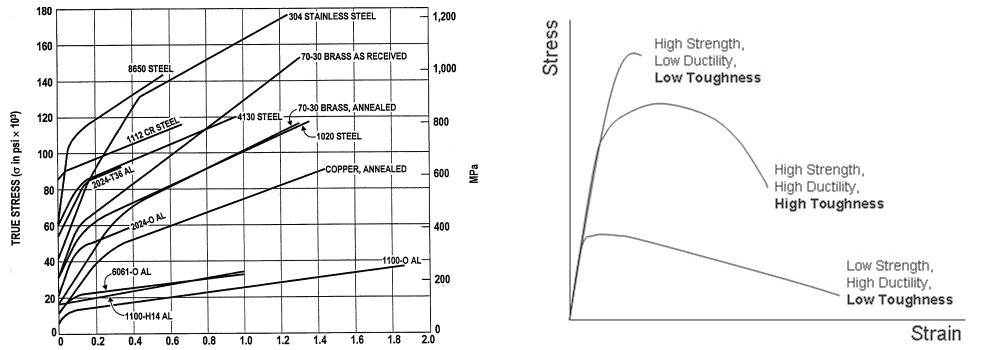• Manufacturing and exporting Hex bolt and Nut
• Manufacturing and exporting Stud bolts and Nuts
• Manufacturing and exporting Sucket (Allen) bolts and Nuts
SELECT LANGUAGE : ENGLISH | PERSIAN
021 55420713 | sales [at] ajaxbolt.com | +989122003350
 HOME ABOUT US PRODUCTS Hex Bolt Sucket Bolt , Allen Bolt Stud bolt Hex Nuts and Heavy Hex Nuts Washer GALLERY CONTACT

# Proof load and Tensile of bolt and nut

Since many technical data sheets associated with bolts and nuts frequently use the terms of tensile, proof load… many of the customers are willing to find out what they are or at least have a superficial understanding of these terms. Therefore, we decided to clarify these terms in the means of both superficial and engineering perspective.

Bolt and nut connections can have different behaviors under axial forces based on the amount of load and their consisting materials. The term Tensile, generally refers to applying axial load to the bolts, but what it is used for in short is the ultimate tensile stress.To understand the meaning of elastic and plastic areas and the behavior of bolt and nut connection under axial load we must first clarify the meaning of tension and strain in simple words.

## Tension

Tension and pressure are two different definitions of one characteristic of force over area. The only difference is that pressure is more commonly used for fluids and gases, while tension is used for solid materials. As mentioned before Tension – illustrated with σ pronounced SIGMA – is defined as normal force over weight.

Tension has the dimension of Newton per square meter also known as Pascal. Since Pascal is a very small tension it is usually used in large as Megapascal or Mpa. In American standard system the dimension of tension is Psi or pound per square inch or in larger scale ksi or kilo pound per square inch. Following is the converting rate between the two systems:

• σ=Fn/A
• 1ksi=6.894Mpa
• 1Mpa=0.145ksi

### Strain

When pulling tension is applied on the bolt connection, it causes elongation. The inflicted elongation can be microscopic in elastic area of visible in plastic area. Strain is defined as the inflicted elongation over the length of bolt and is illustrated with ε which is pronounced Epsilon.

• Ε = ΔL/L
• Strain has no dimension.

### Stress-Strain figure and associated concepts

The stress-strain figure associated with axial load application is presented below### Elastic and Plastic stage

When axial load is applied on the bolt, there first would be a very small elongation which would disappear after omitting the applied force. This area of the figure, where the bolt would return to its initial length after omitting the applied force is called elastic stage or elastic area. Elastic area continues to applying Yield stress. After applying the yield stress bolt enters a new stage called plastic area where any elongation would be permanent and remains even if the load is omitted. Applying further load would increase elongation until the bolt would fracture.

In elastic area the rate of stress and strain remains constant and follows a line, this is while in plastic area the bolt would experience more elongation.

### Proof load and yield stress

Yield stress is the ultimate amount of stress applied to the bolt connection where the bolt would start to deform permanently and would enter the plastic area. Proof load equals 0.85 or 0.9 yield stress and is known as the ultimate safe load where the bolt and nut connection would return to its initial length and would remain in elastic area.

### Tensile and ultimate tensile strength

Ultimate tensile strength is usually used in short term of tensile in most engineering handbooks and define the highest amount of stress , higher than proof load and yield stress, that after this amount the stress would no longer increase, but the elongation would continue to the fracture point. It’s imperative to emphasize that tensile stress does not by any means mean that this amount of stress is allowed to be applied on the bolt and nut connection since tensile stress happens in plastic area, but it only is a scale to define hardness of materials.
Bolt and nut